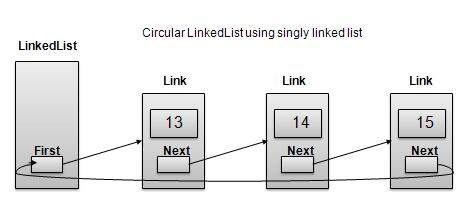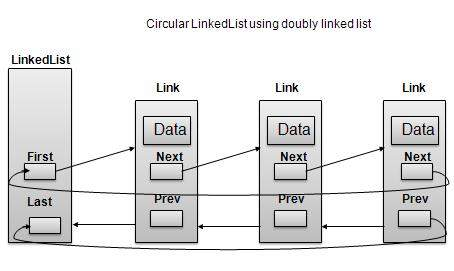# DSA using Java - Circular Linked List

Circular Linked List is a variation of Linked list in which first element points to last element and last element points to first element. Both Singly Linked List and Doubly Linked List can be made into as circular linked list

## Singly Linked List as Circular## Doubly Linked List as CircularAs per above shown illustrations, following are the important points to be considered.

• Last Link'next points to first link of the list in both cases of singly as well as doubly linked list.

• First Link's prev points to the last of the list in case of doubly linked list.

## Basic Operations

Following are the important operations supported by a circular list.

• insert − insert an element in the start of the list.

• delete − insert an element from the start of the list.

• display − display the list.

## length Operation

Following code demonstrate insertion operation at in a circular linked list based on single linked list.

```//insert link at the first location
public void insertFirst(int key, int data){
if (isEmpty()) {
first.next = first;
}
else{
//point it to old first node
//point first to new first node
}
}
```

## Deletion Operation

Following code demonstrate deletion operation at in a circular linked list based on single linked list.

```//delete link at the first location
if(first.next == null){
last = null;
}else {
first.next.prev = null;
}
first = first.next;
}
```

## Display List Operation

Following code demonstrate display list operation in a circular linked list.

```public void display(){
//start from the beginning
//navigate till the end of the list
System.out.print("[ ");
if(first != null){
while(current.next != current){
//print data
current.display();
//move to next item
current = current.next;
System.out.print(" ");
}
}
System.out.print(" ]");
}
```

## Demo

```package com.tutorialspoint.list;

// create an empty linked list
first = null;
}

public boolean isEmpty(){
return first == null;
}

public int length(){
int length = 0;

//if list is empty
if(first == null){
return 0;
}

while(current != first){
length++;
current = current.next;
}
return length;
}

//insert link at the first location
public void insertFirst(int key, int data){
if (isEmpty()) {
first.next = first;
}
else{
//point it to old first node
//point first to new first node
}
}

//delete first item
if(first.next == first){
first = null;
}

//mark next to first link as first
first = first.next;
}

public void display(){

//start from the beginning
//navigate till the end of the list
System.out.print("[ ");
if(first != null){
while(current.next != current){
//print data
current.display();
//move to next item
current = current.next;
System.out.print(" ");
}
}
System.out.print(" ]");
}
}
```

```package com.tutorialspoint.list;

public static void main(String args[]){

list.insertFirst(1, 10);
list.insertFirst(2, 20);
list.insertFirst(3, 30);
list.insertFirst(4, 1);
list.insertFirst(5, 40);
list.insertFirst(6, 56);

System.out.print("\nOriginal List: ");
list.display();
System.out.println("");
while(!list.isEmpty()){
System.out.print("Deleted value:");
temp.display();
System.out.println("");
}
System.out.print("List after deleting all items: ");
list.display();
System.out.println("");
}
}
```

If we compile and run the above program then it would produce following result −

```Original List: [ {6,56} {5,40} {4,1} {3,30} {2,20}  ]
Deleted value:{6,56}
Deleted value:{5,40}
Deleted value:{4,1}
Deleted value:{3,30}
Deleted value:{2,20}
Deleted value:{1,10}
List after deleting all items: [  ]
```